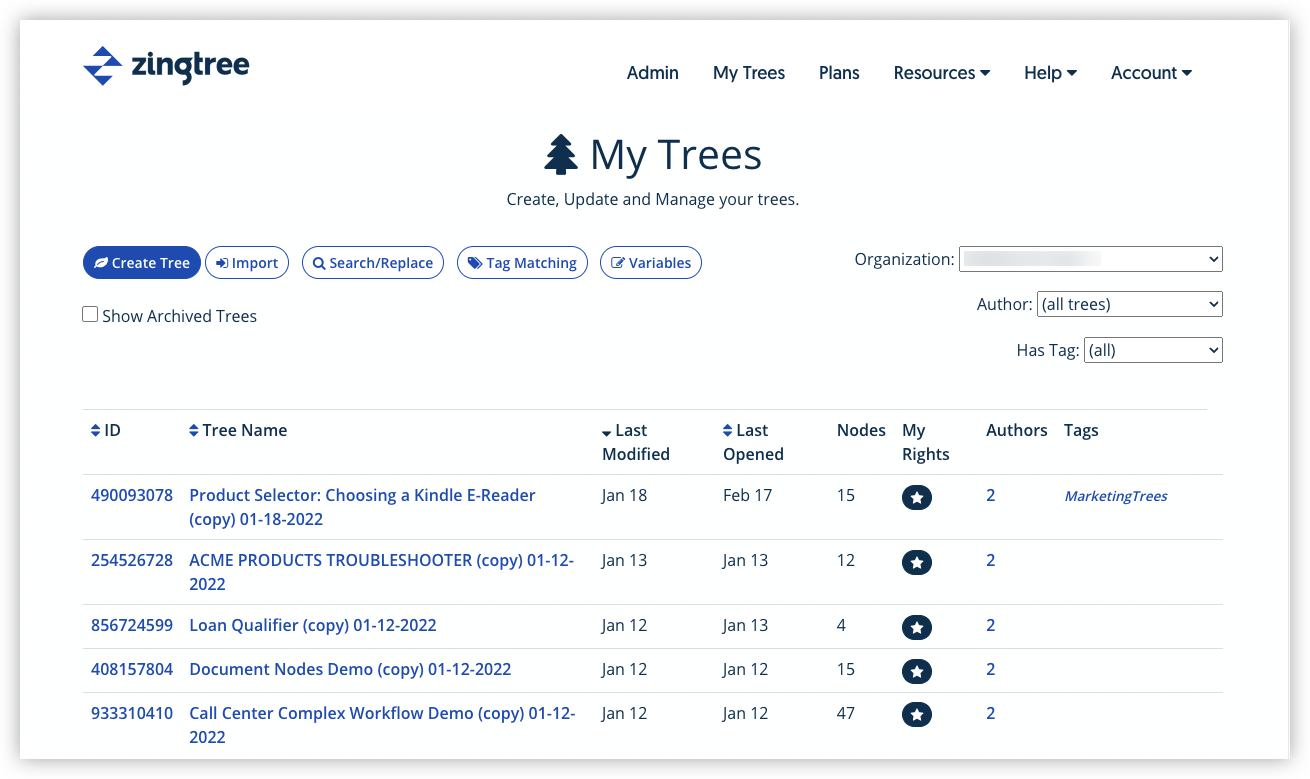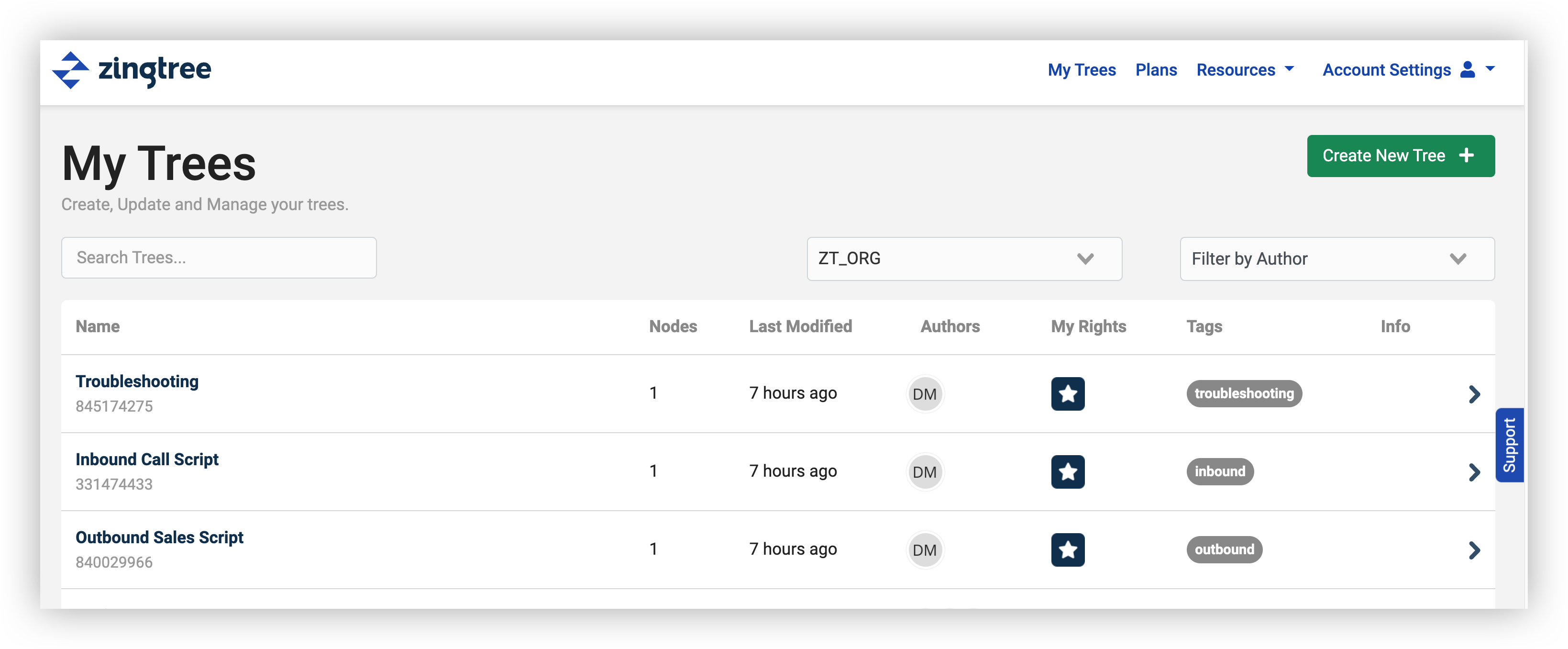# Math Operations

• Updated
• If you’re using Data Entry Fields or Merge Variables to bring data into your decision tree session, you may want to perform math calculations on these variables. Zingtree offers a “Math Webhook” option that lets you assign a new variable to the results of an equation. Here’s how to set it up:

1. Edit the node where you want the new variable (the result of the math operation) to be calculated.
2. Click on the Apps/Webhooks tab.
3. Under Webhook to Call, choose Webhook: Zingtree Math.4. In the Message Data, you enter the equation. You can insert variables (enclosed with # characters), and assign a result to a new variable (no enclosing # characters). In the above example from our Zingtree Math Demo tree, we are assigning the variable area to be equal to the variables width times height.
`area=#width#*#height#`

## Example Calculations

Here are some examples:

result=(#a#+#b#)*3
Set variable result to a plus b, times 3.

area=round (100 * (#radius#^2)*pi)/100
Set the variable area to pi times the radius squared. Then multiply it by 100, round it to an integer, and divide by 100 to make two decimal places only. (“pi” is a built-in constant).

## Reference

These Operations are supported:

 Operation Details Example + Addition 2+3 = 5 – Subtraction 5-3 = 2 * Multiplication 5*3 = 15 / Division 15/3 = 4 ^ Power 5^2 = 25 ( ) parenthesis (2 + 3) * 5 = 25

You can also use these Functions

 Function Details Example exp e raised to the power of x exp(1) = 2.718281828 log natural logarithm log(2.718281828) = 1 sqrt square toot sqrt(25) = 5 round round to nearest integer round(3.14) = 3 floor round down floor (3.14) = 3 ceil round up ceil (3.14) = 4 abs absolute value abs(-2) = 2 sgn sign: -1 if < 0, 1 if > 0 sgn(-20) = -1

Trigonometric functions are also available (sin, cos, tan, etc.)

You can also use the constants pi and .

## Tip

If you are using single-letter variables, then you don’t need to enclose them with # characters. For example, if w and h are the width and height of a rectangle, you can calculate the area with this equation:

area = w*h

## Demo

The Zingtree Demo gallery has an example. You can try it and examine it.

Original Deprecated Math Webhooks (still active, but no longer recommended):

If you’re using Data Entry Fields or Merge Variables to bring data into your decision tree session, you may want to perform basic math operations on these variables. Zingtree offers a stock webhook option that lets you assign a new variable to the results of an addition, subtraction, multiplication or division operation. Here’s how to set it up:

1. Edit the node where you want the new variable (the result of the math operation) to be calculated.
2. Click on the Apps/Webhooks tab.
3. Under Call App or Webhook, choose Webhook: Zingtree Math.4. In the Message Data, you’ll need to provide the operation, the two variables or values to calculate, and a variable name for the result. In the example above, we’re assigning a variable named result to the sum of variables a and b. Variables must be enclosed in # characters.

## Example Calculations

Here are some message examples:

&op=plus&a=5&b=3&result=c
Sets variable c to 5+3

&op=minus&a=#gross#&b=#expenses#&result=net
Sets variable net to the difference of variables gross and expenses.

&op=times&a=#weeks#&b=7&result=days
Sets variable days = weeks * 7.

&op=div&a=#days#&b=7&result=weeks
Sets variable weeks = days / 7.

## Operations Reference

You can use the following in the op= parameter:

• plus
• minus
• times
• div
• If you’re using Data Entry Fields or Merge Variables to bring data into your decision tree session, you may want to perform math calculations on these variables. Zingtree offers a “Math Webhook” option that lets you assign a new variable to the results of an equation. Here’s how to set it up:

1. Edit the node where you want the new variable (the result of the math operation) to be calculated.
2. Click on the Apps/Webhooks tab.
3. Under Call App or Webhook, choose Webhook: Zingtree Math.4. In the Message Data, you enter the equation. You can insert variables (enclosed with # characters), and assign a result to a new variable (no enclosing # characters). In the above example from our Zingtree Math Demo tree, we are assigning the variable area to be equal to the variables width times height.
`area=#width#*#height#`

## Example Calculations

Here are some examples:

result=(#a#+#b#)*3
Set variable result to a plus b, times 3.

area=round (100 * (#radius#^2)*pi)/100
Set the variable area to pi times the radius squared. Then multiply it by 100, round it to an integer, and divide by 100 to make two decimal places only. (“pi” is a built-in constant).

## Reference

These Operations are supported:

 Operation Details Example + Addition 2+3 = 5 – Subtraction 5-3 = 2 * Multiplication 5*3 = 15 / Division 15/3 = 4 ^ Power 5^2 = 25 ( ) parenthesis (2 + 3) * 5 = 25

You can also use these Functions

 Function Details Example exp e raised to the power of x exp(1) = 2.718281828 log natural logarithm log(2.718281828) = 1 sqrt square toot sqrt(25) = 5 round round to nearest integer round(3.14) = 3 floor round down floor (3.14) = 3 ceil round up ceil (3.14) = 4 abs absolute value abs(-2) = 2 sgn sign: -1 if < 0, 1 if > 0 sgn(-20) = -1

Trigonometric functions are also available (sin, cos, tan, etc.)

You can also use the constants pi and .

## Tip

If you are using single letter variables, then you don’t need to enclose them with # characters. For example, if w and h are the width and height of a rectangle, you can calculate the area with this equation:

area = w*h

## Demo

The Zingtree Demo gallery has an example. You can try it and examine it.

Original Deprecated Math Webhooks (still active, but no longer recommended):

If you’re using Data Entry Fields or Merge Variables to bring data into your decision tree session, you may want to perform basic math operations on these variables. Zingtree offers a stock webhook option that lets you assign a new variable to the results of an addition, subtraction, multiplication or division operation. Here’s how to set it up:

1. Edit the node where you want the new variable (the result of the math operation) to be calculated.
2. Click on the Apps/Webhooks tab.
3. Under Call App or Webhook, choose Webhook: Zingtree Math.4. In the Message Data, you’ll need to provide the operation, the two variables or values to calculate, and a variable name for the result. In the example above, we’re assigning a variable named result to the sum of variables a and b. Variables must be enclosed in # characters.

## Example Calculations

Here are some message examples:

&op=plus&a=5&b=3&result=c
Sets variable c to 5+3

&op=minus&a=#gross#&b=#expenses#&result=net
Sets variable net to the difference of variables gross and expenses.

&op=times&a=#weeks#&b=7&result=days
Sets variable days = weeks * 7.

&op=div&a=#days#&b=7&result=weeks
Sets variable weeks = days / 7.

## Operations Reference

You can use the following in the op= parameter:

• plus
• minus
• times
• div
• Throughout 2022 Zingtree will be upgrading customers to our new faster and more modern user experience! Here's how to tell which experience your Zingtree Authors are currently using.

Log in to your Zingtree Author account and navigate to My Trees. How does your current My Trees page look?

#### Zingtree Classic#### New User Experience